# Conditional randomization, standardization, and inverse probability weighting

In a previous post, I began following the developments in Miguel Hernán and James Robins’ soon to be published book, Causal Inference. There I gave an overview of the first topics they cover, namely potential outcomes, causal effects, and randomization. In this post I’ll continue, with some personal notes on the remaining parts of Chapter 2 of their book, on conditional randomization, standardization, and inverse probability weighting.

Conditional randomization
In simple randomization in a trial, each unit or individual is randomized to one of (usually) two groups, entirely randomly. I’ll refer to the two groups as the control and treatment group. In a conditionally randomized trial or experiment, we first stratify or split the sample according to a baseline stratification variable (or variables)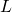. In each of these strata, we randomize individuals to the control and treatment groups, and furthermore we may choose to use different randomization probabilities in the different strata. For example ifis binary, we may randomized individuals with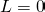to the treatment group with probability 0.75, but in those with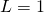we may randomize to the treatment group with probability 0.25 (for example).

For a regular, marginally randomized trial, Hernán and Robins make the important connection that this is equivalent to the potential outcomes being missing completely at random. In a conditionally randomized trial, the potential outcomes are instead missing at random, meaning that the probability that (say) the potential outcome under treatment is observed is independent of that potential outcome value, conditional on the variable(s).

At this point you may reasonably wonder why we would use such a randomization scheme! Well in reality, such a conditional randomization scheme would not often be used. As we shall see in the following, the randomization scheme makes things somewhat more complicated if we want to draw inferences about causal effects in the total (unstratified) population. Why then are we talking about conditional randomization? Because when we come to observational studies, where treatment is not randomized, to make progress we will pretend that the observational study is like a conditionally randomized trial. Here we will assume that whether or not an individual receives the treatment (rather than the control) is random, conditional on confounders.

If we have data from a conditionally randomized trial, how can we estimate population causal effects?

Standardization
The first approach described by Hernán and Robins is standardization. Suppose we are interested in estimating the average causal effect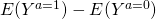(we could be interested in another causal effect measure, e.g. the causal risk ratio). To estimate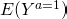, we can use the law of total expectation to write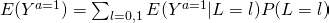To estimate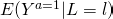, we can use the observed risk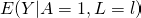among those with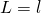and who were randomized to treatment. That these two are identical holds because within each level of, we have a regular, marginally randomized trial (albeit with a randomization probability that in general need not equal 0.5). The probability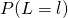can then be easily estimated by the sample proportion of individuals who have.

Inverse probability weighting
To estimate the potential outcome mean under treatment,, we might, as in a regular marginally randomized trial, consider using the mean of the observed outcome among those randomized treatment,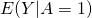. This however would be a biased estimate. Why? Because we have randomized to treatment with different probabilities depending on the value of the variable, those randomized to treatment have a different distribution ofto those randomized to control. Ifaffects the outcome too, we would obtain a biased estimate due to confounding by.

How then can we validly estimate the mean outcome under treatment? The second approach described by Hernán and Robins is inverse probability weighting. In the inverse probability weighting approach, we can calculate the mean of the observed outcomes among those who were treated, but weighting by the reciprocal of the probability that each of these individuals would have been treated. Earlier, we said that in our conditionally randomized trial, we might randomize those withto the treatment group with probability 0.75, but in those withwe may randomize to the treatment group with probability 0.25. In this case, when calculating the mean of the observed outcomes to estimate, we would weight those withby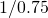and those withby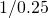. In essence, by inverse probability weighting, we assign weights to those who were treated such that their distribution ofis the same as the population distribution of. By doing this we obtain a consistent estimate of the population mean potential outcome under treatment,.

We can then do the same among those randomized to control, in order to estimate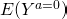, where here we weight by the reciprocal of the probability of being randomized to control. This weighting ensures that the weighted sample of control individuals have a distribution ofwhich matches the population distribution of. Now we have valid estimates ofand, we can calculate the causal effect measure of interest, e.g..

### You may also be interested in:

This site uses Akismet to reduce spam. Learn how your comment data is processed.